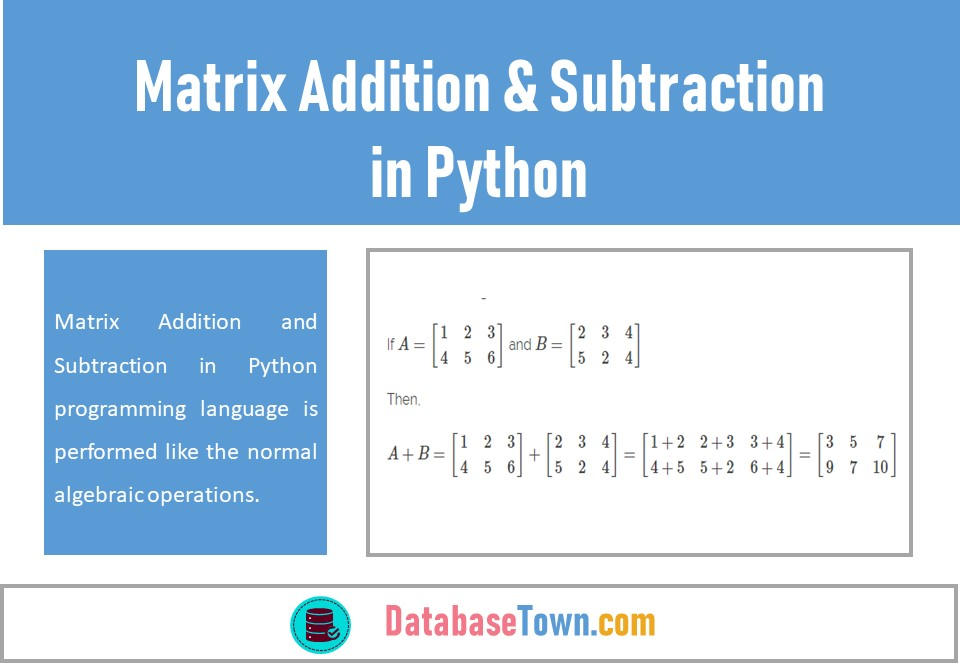# Matrix Addition and Subtraction in Python

Matrix Addition and Subtraction in Python programming language is performed like the normal algebraic operations.

Before discussing these operations, it is necessary to introduce a bit about Algebra which has been taken from the Arabic word Al-Jabar, afterward, this word turned into Algebra.

Algebra is a branch of Mathematics that provides an easy solution to many complex mathematical problems especially when quantity is represented by a sign without any arithmetical value.

## Addition of Matrices

Generally, the addition of two matrices A and B is possible if they have the same orders. The addition of two matrices A and B is denoted by A + B. Mathematical example of addition of two matrices is given here.

If $$\displaystyle A=\left[ {\begin{array}{*{20}{c}} 1 & 2 & 3 \\ 4 & 5 & 6 \end{array}} \right]$$ and $$\displaystyle B=\left[ {\begin{array}{*{20}{c}} 2 & 3 & 4 \\ 5 & 2 & 4 \end{array}} \right]$$

Then,

$$\displaystyle A+B=\left[ {\begin{array}{*{20}{c}} 1 & 2 & 3 \\ 4 & 5 & 6 \end{array}} \right]+\left[ {\begin{array}{*{20}{c}} 2 & 3 & 4 \\ 5 & 2 & 4 \end{array}} \right]$$ $$\displaystyle =\left[ {\begin{array}{*{20}{c}} {1+2} & {2+3} & {3+4} \\ {4+5} & {5+2} & {6+4} \end{array}} \right]=\left[ {\begin{array}{*{20}{c}} 3 & 5 & 7 \\ 9 & 7 & {10} \end{array}} \right]$$

Let’s start a practical example of addition of two matrices in python…

First, we import the relevant libraries in Jupyter Notebook as shown below,

import numpy as np

We use NumPy, a library for the python programming that allows us to work with multidimensional arrays and matrices along with a large collection of high-level mathematical functions to operate on these arrays.

Now we perform the addition of two matrices A and B like this,

Subtraction of Matrices

The subtraction of one matrix from another matrix will be possible if they have the same orders and subtraction of two matrices A and B is denoted by A – B. Mathematical example of subtraction to two matrices is given below

If

$$\displaystyle A=\left[ {\begin{array}{*{20}{c}} 1 & 2 & 3 \\ 5 & 6 & 7 \end{array}} \right]$$ and $$\displaystyle B=\left[ {\begin{array}{*{20}{c}} 2 & 5 & 3 \\ 4 & 1 & 8 \end{array}} \right]$$

Then,

$$\displaystyle A-B=\left[ {\begin{array}{*{20}{c}} 1 & 2 & 3 \\ 5 & 6 & 7 \end{array}} \right]-\left[ {\begin{array}{*{20}{c}} 2 & 5 & 3 \\ 4 & 1 & 8 \end{array}} \right]$$

$$\displaystyle =\left[ {\begin{array}{*{20}{c}} {1-2} & {2-5} & {3-3} \\ {5-4} & {6-1} & {7-8} \end{array}} \right]=\left[ {\begin{array}{*{20}{c}} {-1} & {-3} & 0 \\ 1 & 5 & {-1} \end{array}} \right]$$

Let’s start a practical example of subtraction of two matrices in python. We have already imported the relevant libraries in Jupyter Notebook as mentioned above, therefore, now we perform the subtraction of two matrices A and B like this,

Let’s see an example of addition of two vectors but we must care about the length of these two vectors that should be same.

An example of subtraction of two vectors having same length is given below,

Related post: Linear Algebra for Data Science